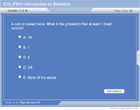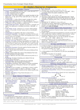How to Learn in 24 Hours?The Rapid Learning Movie

 Need Help? M-F: 9am-5pm(PST): Toll-Free: (877) RAPID-10 US Direct: (714) 692-2900 Int'l: 001-714-692-2900 24/7 Online Technical Support: The Rapid Support Center Secure Online Order:Got Questions? Frequently Asked Questions
 Need Proof? Testimonials by Our Users
 Trustlink is a Better Business Bureau Program. Rapid Learning Center is a fivr-star business. External TrustLink Reviews

 Rapid Learning Courses: MCAT in 24 Hours (2015-16) USMLE in 24 Hours (Boards) Chemistry in 24 Hours Biology in 24 Hours Physics in 24 Hours Mathematics in 24 Hours Psychology in 24 Hours SAT in 24 Hours ACT in 24 Hours AP in 24 Hours CLEP in 24 Hours DAT in 24 Hours (Dental) OAT in 24 Hours (Optometry) PCAT in 24 Hours (Pharmacy) Nursing Entrance Exams Certification in 24 Hours eBook - Survival Kits Audiobooks (MP3)

 Tell-A-Friend: Have friends taking science and math courses too? Tell them about our rapid learning system.Home »  Mathematics »  Introductory Statistics

Introduction to Introductory Statistics

 Topic Review on "Title": Statistical procedures used to summarize, organize, and simplify data. Population: An entire collection of data. Scores : Each collection of data Total scores: N is the total number of scores of population Sample: A subset of population. Random: Chosen in a way to allow each member of the population an equal chance of being selected. Response Rate: The proportion of people who respond to a survey. Statistics Study Tips: Think statistically and try to apply in day to day life. Setting up each segments of the problem. Rather than memorize the formula, think about how to derive the formula and identify its parts. Try step by step approach. Draw a diagram if needed.  Identify all given information. Select appropriate formula(s) that incorporate what you know and what you want to find. Measures of Central tendency are measures of the location of the middle or the center of a distribution- (Mean, Median, Mode, Mid-range). Measures of Variability describes in an exact quantitative measure, how spread out/clustered together the scores are-(Range, Variance, Standard deviation).

Rapid Study Kit for "Title":
 Flash Movie Flash Game Flash Card Core Concept Tutorial Problem Solving Drill Review Cheat Sheet"Title" Tutorial Summary : The purpose and scope of introductory statistics is discussed in this tutorial with the introduction of relations and their usage. After finishing this course, you will be able to understand what is data and the relationships among the data, how to generate data, the random behavior of the data, and the statistical inference techniques with some topics in inference. This tutorial gives an overview of what to look forward to in this course. It also summarizes the most important units and principles in this introductory statistics course. It is a strong tool to use for anyone who is unfamiliar or is learning introductory statistics for the very first time.

 Tutorial Features: Specific Tutorial Features: Understanding Data Understanding Relationships Generating Data Random Behavior Statistical Inference Topics in Inference Series Features: Concept map showing inter-connections of concepts introduced. Definition slides introduce terms as they are needed. Examples given throughout to illustrate how the concepts apply. A concise summary is given at the conclusion of the tutorial.

 "Title" Topic List: Statistics Study Tips Problem Solving Skills Measures of Central Tendency – Mean, Median, Mode, Midrange Measures of Variability – Range, Variance, Standard Deviation Probability Frequency Distribution – Categorical, Histogram, Bar chart, Stem-and- Leaf Plot Testing Hypothesis – Alternative, Null, Type I & Type II Errors, Limit Theorem Probability Distribution – Uniform, Normal, Exponential

See all 24 lessons in Introductory Statistics, including concept tutorials, problem drills and cheat sheets:
Teach Yourself Introductory Statistics Visually in 24 Hours